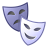# SSI as i2s master with 16 bit audio

キャンセル

もしかして:

## SSI as i2s master with 16 bit audio

ソリューションへジャンプ
923件の閲覧回数Contributor III

It it possible to configure the SSI in i2s master mode while playing or recording 16 bit audio?

According to the reference manual for i.MX6, the "word length is fixed to 32 in I2S Master mode". Is there any way to work around this limitation? Or is it simply impossible to use master mode if one wants 16 bit?

ラベル(2)

• ### Multimedia

タグ(3)
1 解決策
661件の閲覧回数Contributor III

The following workaround seems to work:  Set SSIx_SCR.I2S_MODE to 0 (normal mode) instead of 1 (I2S master mode). This has been tested in loopback mode as well as with an external codec. Driver was initialised with the following register settings:

SCR = 0x00001119
SSIEN = 0x1
TE = 0x0
RE = 0x0
NET = 0x1
SYN = 0x1
I2S_MODE = 0x0        <<<------ Normal mode instead of i2s master mode
SYS_CLK_EN = 0x0
TCH_EN = 0x1
CLK_IST = 0x0
TFR_CLK_DIS = 0x0
RFR_CLK_DIS = 0x0
SYNC_TX_FS = 0x1
SISR = 0x00003003
SIER = 0x00280000
STCR = 0x000003ED
TEFS = 0x1
TFSL = 0x0
TFSI = 0x1
TSCKP = 0x1
TSHFD = 0x0
TXDIR = 0x1
TFDIR = 0x1
TFEN0 = 0x1
TFEN1 = 0x1
TXBIT0 = 0x1
SRCR = 0x0000078D
REFS = 0x1
RFSL = 0x0
RFSI = 0x1
RSCKP = 0x1
RSHFD = 0x0
RXDIR = 0x0
RFDIR = 0x0
RFEN0 = 0x1
RFEN1 = 0x1
RXBIT0 = 0x1
RXEXT = 0x1
STCCR = 0x0000E116
PM7_PM0 = 0x16
DC4_DC0 = 0x1
WL3_WL0 = 0x7
PSR = 0x0
DIV2 = 0x0
SRCCR = 0x00040000
SFCSR = 0x00880088
TFWM0 = 0x8
RFWM0 = 0x8
TFCNT0 = 0x0
RFCNT0 = 0x0
TFWM1 = 0x8
RFWM1 = 0x8
TFCNT1 = 0x0
RFCNT1 = 0x0
SACNT = 0x00000000
SACDAT = 0x00000000
SATAG = 0x00000000
STMSK = 0xFFFFFFFC
SRMSK = 0xFFFFFFFC

3 返答（返信）
662件の閲覧回数Contributor III

The following workaround seems to work:  Set SSIx_SCR.I2S_MODE to 0 (normal mode) instead of 1 (I2S master mode). This has been tested in loopback mode as well as with an external codec. Driver was initialised with the following register settings:

SCR = 0x00001119
SSIEN = 0x1
TE = 0x0
RE = 0x0
NET = 0x1
SYN = 0x1
I2S_MODE = 0x0        <<<------ Normal mode instead of i2s master mode
SYS_CLK_EN = 0x0
TCH_EN = 0x1
CLK_IST = 0x0
TFR_CLK_DIS = 0x0
RFR_CLK_DIS = 0x0
SYNC_TX_FS = 0x1
SISR = 0x00003003
SIER = 0x00280000
STCR = 0x000003ED
TEFS = 0x1
TFSL = 0x0
TFSI = 0x1
TSCKP = 0x1
TSHFD = 0x0
TXDIR = 0x1
TFDIR = 0x1
TFEN0 = 0x1
TFEN1 = 0x1
TXBIT0 = 0x1
SRCR = 0x0000078D
REFS = 0x1
RFSL = 0x0
RFSI = 0x1
RSCKP = 0x1
RSHFD = 0x0
RXDIR = 0x0
RFDIR = 0x0
RFEN0 = 0x1
RFEN1 = 0x1
RXBIT0 = 0x1
RXEXT = 0x1
STCCR = 0x0000E116
PM7_PM0 = 0x16
DC4_DC0 = 0x1
WL3_WL0 = 0x7
PSR = 0x0
DIV2 = 0x0
SRCCR = 0x00040000
SFCSR = 0x00880088
TFWM0 = 0x8
RFWM0 = 0x8
TFCNT0 = 0x0
RFCNT0 = 0x0
TFWM1 = 0x8
RFWM1 = 0x8
TFCNT1 = 0x0
RFCNT1 = 0x0
SACNT = 0x00000000
SACDAT = 0x00000000
SATAG = 0x00000000
STMSK = 0xFFFFFFFC
SRMSK = 0xFFFFFFFC

661件の閲覧回数NXP Employee

Hi Frederik

"word length is fixed to 32 in I2S Master mode" is SSI hardware limitation,

unfortunately there is no way to work around this limitation.

Best regards
igor
-----------------------------------------------------------------------------------------------------------------------
-----------------------------------------------------------------------------------------------------------------------

661件の閲覧回数Contributor III

Hi Igor, and thanks for the reply!

Since posting this question, I've tried a potential workaround. Instead of setting SSIx_SCR.I2S_MODE to 1 (I2S master mode) I set it to 0 (normal mode). All other register settings are the same as described in the i.MX 6 reference manual chapter 61.8.1.4 "I2S Mode" when configuring I2s master mode, including those bits that would be internally overridden had I2S master mode been selected in SSIx_SCR.I2S_MODE.

The samples generated are now 16 bit instead of 32, as seen by oscilloscope. I've also done loopback tests where the received samples are the same as the sent samples. I havent't tried to communicate with a real audio codec yet.

It would be great if you could look at this workaround and see if there is any flaw in it, or if it is safe.

Best regards,

Fredrik